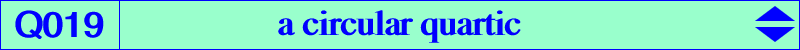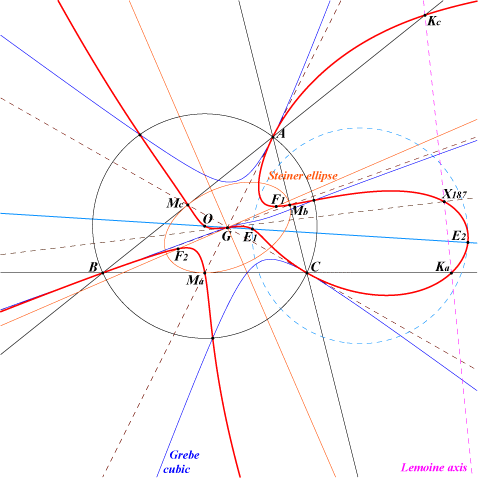too complicated to be written here. Click on the link to download a text file.X(2), X(3), X(187), X(3413), X(3414), X(5000), X(5001) Ma, Mb, Mc : midpoints of ABC Ka, Kb, Kc : feet of the Lemoine axis (centers of the apollonian circles) four foci of the Steiner in-ellipse (two real F1, F2) common points of the circumcircle and the Grebe cubic K102 i.e. vertices of the Grebe triangle. See also Table 57 imaginary foci of the Brocard ellipse i.e. common points of the Brocard axis and the Kiepert hyperbola anti-points, see Table 77Notes : • X(3413), X(3414) are the points at infinity of the Kiepert hyperbola. • E1, E2 on the Euler line are X(5000), X(5001). See Table 55.Q019 is a circular quartic with two real asymptotes parallel at X(625) to those of the Kiepert hyperbola. Its singular focus is X(187), the inverse of K in the circumcircle. The tangents at A, B, C are the medians. G is a flex with inflexional tangent the line G-X(187). Q019 meets the Euler line at O, H and two (not always real) other points E1, E2 on the circle centered at X(468) orthogonal to the circumcircle. Locus properties Let P be a point and let K', K" be the Lemoine points of the pedal and antipedal triangles of P respectively. P, K', K" are collinear if and only if P lies on the quartic Q019 or on the line at infinity. P, G and the centroid of the circumcevian triangle of P are collinear if and only if P lies on Q019 (together with the circumcircle and the line at infinity). Compare Q019 and Q021, Q024. Let M be a point, M* its isogonal conjugate, M' its inverse in the circumcircle. The points M*, M' and K (Lemoine point) are collinear if and only if M lies on Q019. See Q064 for an analogous quartic when K is replaced by H. Q019 is the locus of the centers of central focal circum-cubics passing through G. See K065 (center G) and K435 (center X187) for instance. See the related curves K026 and Q089. *** The isogonal transform of Q019 is Q094. See also Table 45 and the similar circular quartics Q115, Q116, Q117, Q118.